# microsoft-interview-questionsMedian of Two Sorted Arrays

There are two sorted arrays A and B of size n each, write a program to find the median of these two sorted arrays obtained after merging (the new merged array will be an array of length 2n). The median of a sorted array of size n is defined as the middle element when n is odd and the average of the middle two elements when n is even.Pair sum in an array

Given an array of n integers and a target number, write a program to find whether a pair sum exists in the array or not. In other words, we need to check for a pair of elements in the array that sum exactly to the target value. Assume that all elements are distinct. Note: This is an excellent problem to learn problem solving using two pointers and hash table.Given an array of n elements, write a program to find the maximum subarray sum. A subarray of array X[] is a contiguous segment from X[i] to X[j], where 0 <= i <= j <= n-1. Note: Max subarray sum is an excellent problem to learn problem-solving using the divide and conquer approach, dynamic programming, and single loop (kadane's algorithm).Flood Fill Algorithm

The flood fill problem is a way to fill a region of connected pixels with a new colour, starting from a given pixel in an image. You are given an image represented by an m x n grid of integers, where the image[i] represents the pixel value at position (i, j). You are also given the coordinates of a starting pixel (x, y) and a new colour to use for the flood fill operation.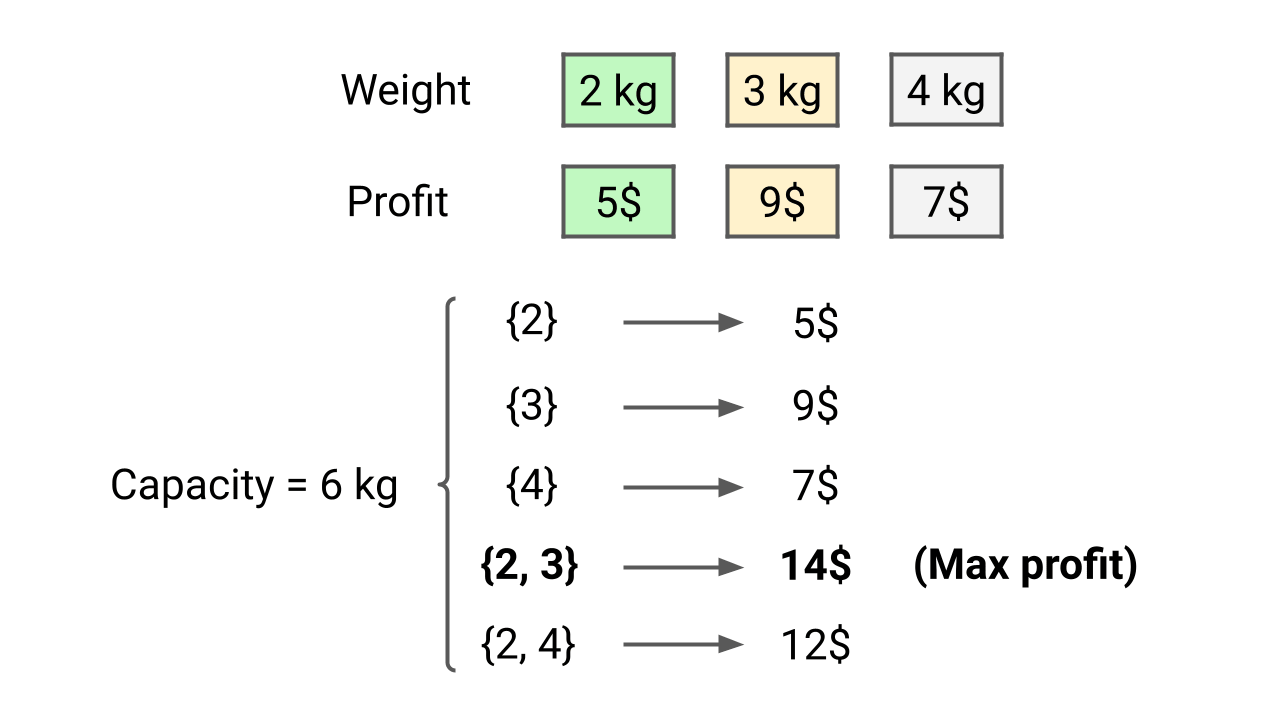0-1 Knapsack Problem

We are given n items, where each item has a weight and a profit associated with it. We are also given a knapsack with a capacity of C (the knapsack can hold a maximum weight of C). Write a program to determine the maximum profit that can be obtained by selecting a subset of items without exceeding the knapsack's capacity.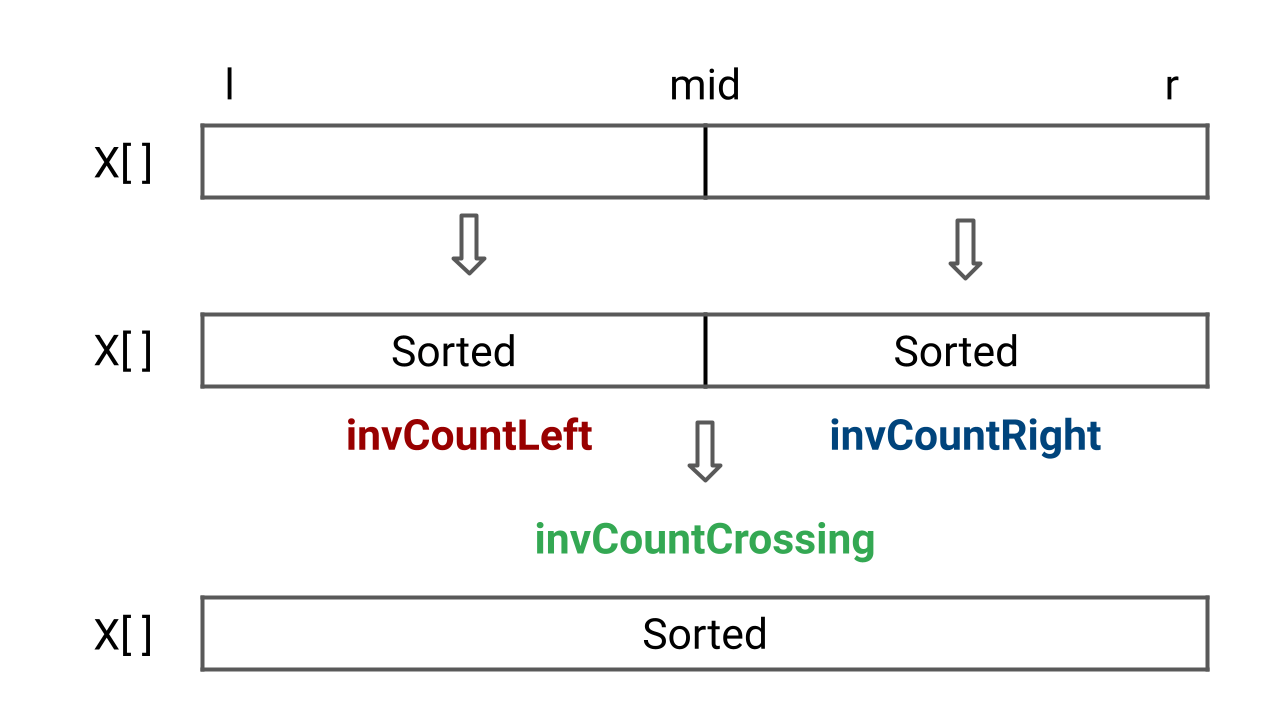Count Inversions of an Array

Given an array of n integers, write a program to find the total number of inversion counts. An inversion occurs when there are two elements in the array such that i < j and X[i] > X[j]. Here, the pair (i, j) is called an inversion of X[], and the inversion count represents the count of such inversions present in the array.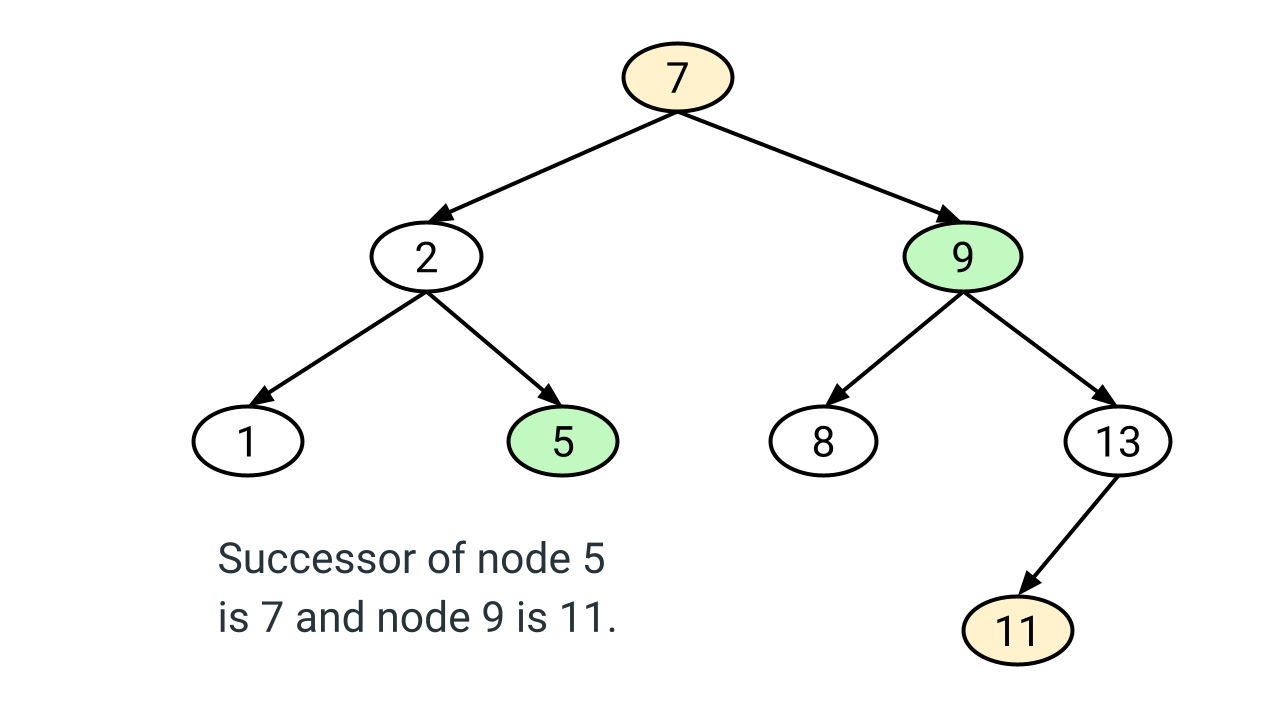Inorder Successor of a Node in Binary Search Tree (BST)

Given a node x and the root of a binary search tree, write a program to find the in-order successor of node x. The in-order successor of node x is a node that will be visited just after node x during an in-order traversal. In other words, the in-order successor of a node x is the node with the smallest key greater than x->key.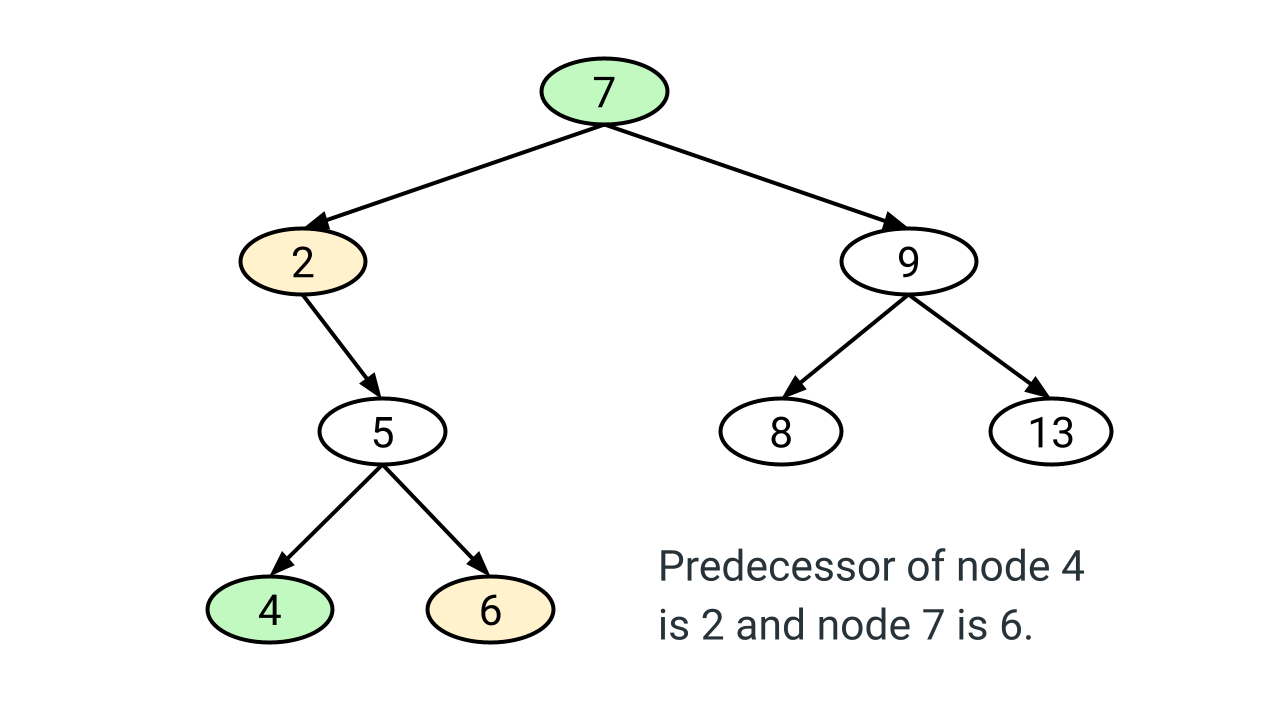Inorder Predecessor of a Node in Binary Search Tree (BST)

Write a program to find the in-order predecessor of a given node x in a binary search tree (BST). An in-order predecessor of node x is the node that will be visited just before node x in the in-order traversal. If the node x is visited first (leftmost node in BST) then the predecessor of node x is NULL.Quicksort Algorithm

Quicksort algorithm is often the best choice for sorting because it works efficiently on average O(nlogn) time complexity. It is also one of the best algorithms to learn divide and conquer approach. In this blog, you will learn: 1) How quick sort works? 2) How to choose a good pivot? 3) Best, worst, and average-case analysis 4) Space complexity and properties of quicksort.Merge Sort Algorithm

Merge sort is one of the fastest comparison-based sorting algorithms, which works on the idea of a divide and conquer approach. The worst and best-case time complexity of the merge sort is O(nlogn), and the space complexity is O(n). It is also one of the best algorithms for sorting linked lists and learning the design and analysis of recursive algorithms.Find Minimum and Maximum Element in an Array

Given an array X[] of size n, write a program to find the maximum and minimum elements while making the minimum number of comparisons. This is an excellent question to learn problem-solving using a single loop and divide and conquer approach. In the efficient single-loop solution, we increment the loop by two to optimize the comparison count.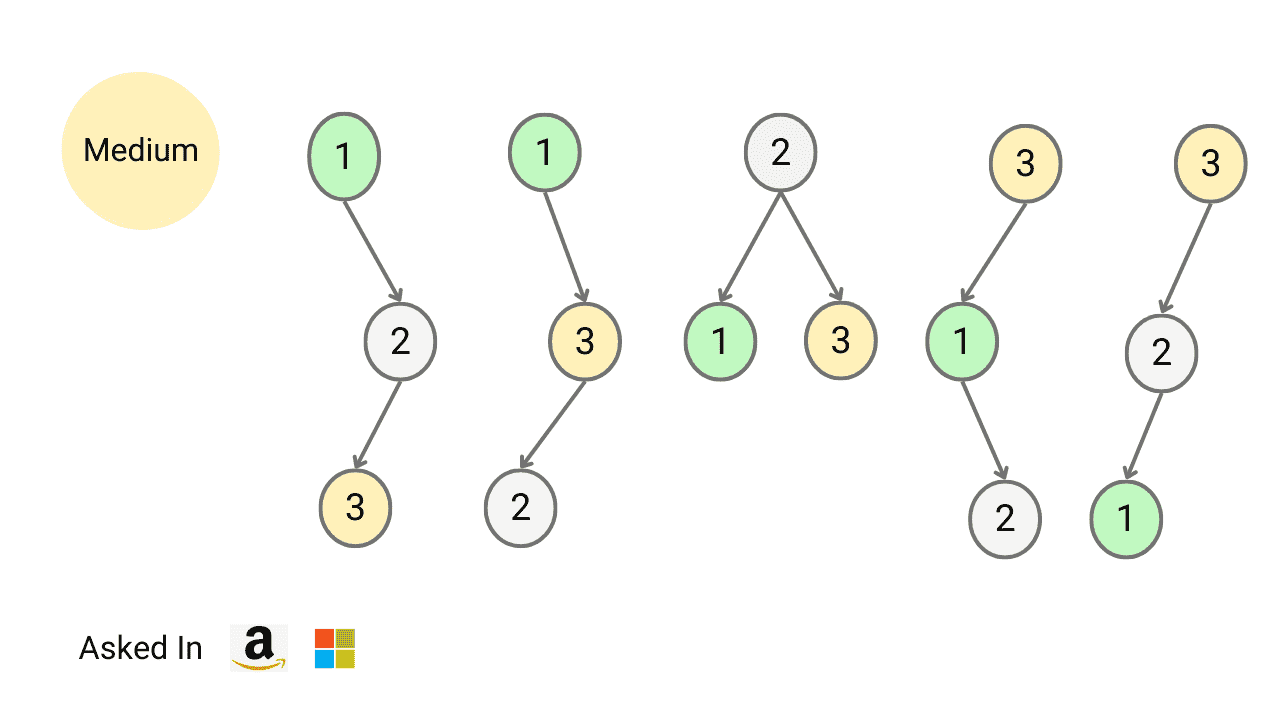Count Total Number of Unique Binary Search Trees with n Keys

Write a program to find the number of structurally unique binary search trees (BSTs) that have exactly n nodes, where each node has a unique integer key ranging from 1 to n. In other words, we need to determine the count of all possible BSTs that can be formed using n distinct keys.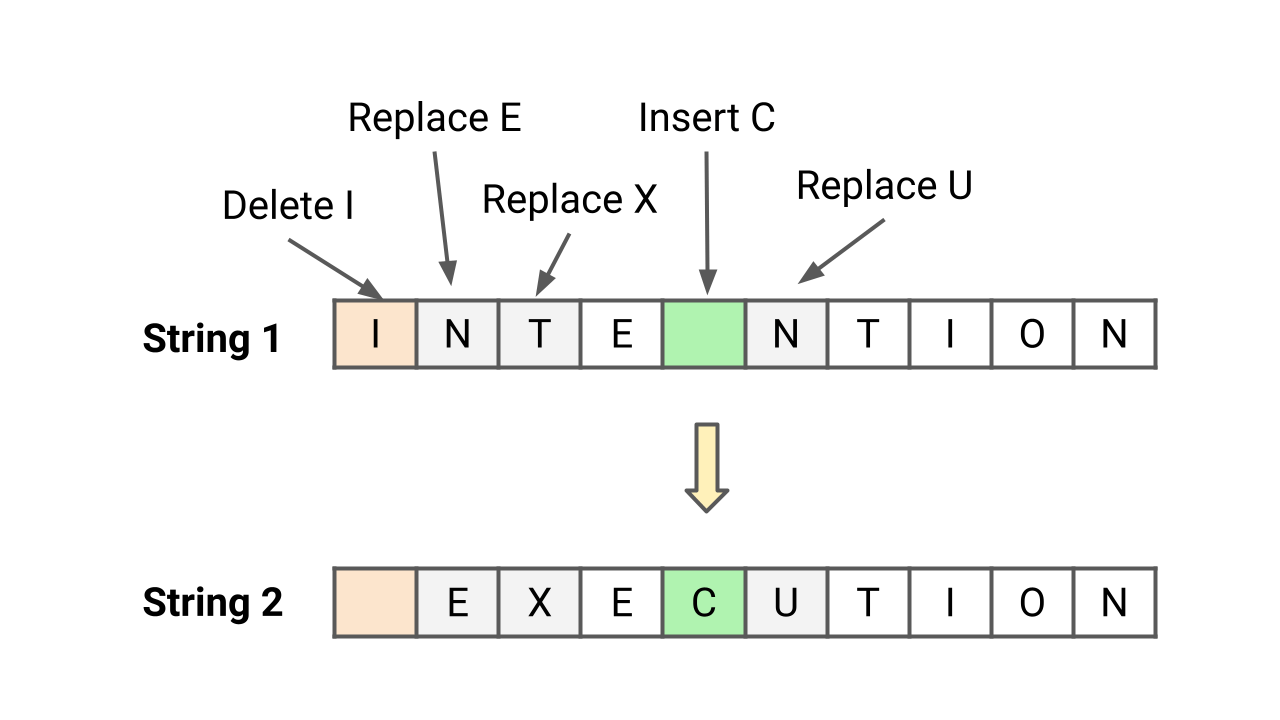Edit Distance (Levenshtein Distance) Problem

Write a program to find the minimum number of operations required to convert string X to string Y. You have the following three operations permitted on a string: 1) Insert a character 2) Delete a character 3) Replace a character. The edit distance between two strings is the minimum number of operations (insertions, deletions, or substitutions of characters) required to transform one string into the other.Counting Sort Algorithm

Counting sort is a stable sorting algorithm that works in O(n) time and space complexity when input are integers in the range 0 to k and k = O(n). Instead of comparison, counting sort uses array indexing to determine position of elements. For each element x, it counts values less than x and places x directly into its correct position in the sorted array.Detect Loop or Cycle in Linked List

Given the head of a linked list, write a program to find if linked list has a cycle or not. Return true if there is a cycle or loop in the linked list. Otherwise, return false. A linked list with cycle causes iteration to fail because the iteration will never reach the end. So, detecting a linked list loop is important before applying an iterative approach.Implement Stack using Queues

Write a program to implement a stack using queues. We must use queue operations like enqueue, dequeue, front, size to implement stack operations like push, pop, and top. We have discussed three approaches: 1) Using two queues: O(n) pop and O(1) push 2) Using two queues: O(1) pop and O(n) push 3) Using one queue: O(1) pop and O(n) push.Implement Queue Using Stacks

Write a program to implement queue using stack. We should use stack operations like push, pop, top, size, and isEmpty for implementing queue operations like enqueue, dequeue, and front. In this blog, we have discussed two approaches for implementing queue using two stacks: 1) Dequeue O(1) and Enqueue O(n) 2) Enqueue O(1) and Dequeue O(1).Kth Smallest Element in an Array

Given an array and a positive integer k, write a program to find the kth smallest element in the array. This is an excellent problem to learn problem-solving using the max and min heap data structures. The quick-select approach (divide and conquer) is also worth exploring because it helps to optimize time complexity to O(n) on average.Minimum Coin Change Problem

Suppose we want to make a change for a given value K of cents, and we have an infinite supply of each of coin[ ] = [C​1​​, C​2​​, …, C​m​​] valued coins. Write a program to find the minimum number of coins required to make the change. Note: This is an excellent counting problem to learn problem solving using dynamic programming approach.Find Next Greater Element

Given an array, find the next greater element for every element in the array (NGE). The next greatest element for an element is the first largest element on the right side. For elements for which no next largest element exists, consider the next greater element as -1. We have discussed two stack-based solutions: 1) Traversing from left to right, 2) Traversing from right to left.Remove Duplicates from Sorted Array

Given a sorted array, write a program to remove duplicates from the array. We need to remove repeated elements so that there is a single occurrence of each element and return the length of the array containing unique elements. Note: This is an excellent problem to learn the fast and slow pointers approach. We have discussed two O(n) time in-place solutions.Row With Max 1s

Given a boolean 2D array, where each row is sorted. Find the row with the maximum number of 1s. This is an excellent matrix problem that can be solved in linear time complexity. The best part is — we are using the sorted order property and nested loops to improve the solution over the binary search approach.Find First and Last Position of Element in Sorted Array

Given an array of integers sorted in ascending order, the program should find the first and last occurrence of a given element. The goal is to design an algorithm with O(log n) time complexity. Note that this is an excellent question to learn problem-solving using binary search. Here, the binary search needs to be applied twice to solve this problem.Majority Element

You are given an array X[] of n elements, write a program to find majority element in an array. A majority element is an element that appears more than n/2 times, so there is at most one such element. Assume that array is non-empty and majority element always exists in the array. Note: This is an excellent problem to learn various approaches.Maximum Difference in an Array

Given an array A[] of n integers, find out the maximum difference between any two elements such that the larger element appears after the smaller element. In other words, we need to find max(A[j] - A[i]), where A[j] > A[i] and j > i. Note: This is an excellent problem to learn problem-solving using divide and conquer and a single loop.Find Triplets with Zero Sum

Given an array X[] of n distinct elements, write a program to find all the unique triplets in the array whose sum is equal to zero. For example, if triplets with zero sum in the array are (X[i], X[j], X[k]), then X[i] + X[j] + X[k] = 0. Hint: This is an excellent problem to learn problem-solving and optimization using hashing and two pointers approach.Longest Substring Without Repeating Characters

Given a string S, write a program to find the length of longest substring without repeating characters. The substring is a continuous subpart of the string and we need to return the largest substring which has all unique characters. Note: This is an excellent problem to learn problem solving and time complexity optimization using sliding window approach.Trapping Rain Water

Given n non-negative integers representing an elevation map where the width of each bar is 1. Write a program to compute how much water it can trap after raining. This is a famous interview problem to learn time and space complexity optimization using various approaches. Two pointers approach provides an efficient solution using O(n) time and O(1) space.Sort an Array of 0s, 1s and 2s (Dutch National Flag Problem)

Given an array consisting of 0s, 1s, and 2s, write a program to sort this array of 0, 1, and 2 in ascending order. We need to sort the array in O(n) time complexity without using sorting algorithms or extra space. Note: This is a variation of the Dutch national flag problem and an excellent problem to learn problem solving using three pointers.Search in a row wise and column wise sorted matrix

You have given row-wise and column-wise sorted 2d matrix and integer k, write a program to search k in 2d matrix, i.e. find whether k is present or not. Each row is sorted from left to right, and the first integer of each row is greater than the last integer of the previous row. Note: This is an excellent problem to learn problem solving binary search in a 2d matrix.Find Maximum Element in a Bitonic Array

You are given an array of n integers that is first increasing and then decreasing. Write a program to find the maximum value in the array. Our goal should be to solve this problem using O(logn) time complexity. Note: This is an excellent problem to learn problem solving using binary search, where we modify binary search to get an efficient solution.Number of Buildings Facing Sun

Given an input array height[] which represents the heights of buildings, write a program to count the number of buildings facing the sunset. Assume that the heights of buildings are distinct. Insight: Here all buildings facing sunlight will be in increasing order. Note: This is an excellent problem to learn problem-solving using a single loop.FizzBuzz Problem

Given an integer n, write a program to return string representation of numbers from 1 to n. If number is divisible by 3, we store “Fizz”. If number is divisible by 5, we store “Buzz”. If number is divisible by both 3 and 5, we store “FizzBuzz”. Hint: This is an excellent puzzle to learn coding concepts like conditional statements, loops, etc.Write a program to find intersection of two sorted linked lists and return the head pointer of the new linked list. Here head pointers of both sorted linked lists are given as input and we shouldn’t do any changes in the structure to generate the output. Note: This is an excellent problem to learn problem-solving using recursion and two pointers in a linked list.Check whether two strings are anagram or not

Given two strings, str1 and str2, of size m and n, write a program to check whether two strings are an anagram of each other or not. A string str1 is an anagram of str2 if characters of str1 can be rearranged to form str2. Note: This is an excellent string problem to learn problem-solving and optimization using direct address table.Rotate Matrix by 90 Degrees

Given an n x n 2D matrix, write a program to rotate the matrix by 90 degrees in the anticlockwise direction. The program should rotate the matrix 90 degrees without using extra space. In other words, we have to perform the rotation by modifying the 2D matrix directly. Note that this is an excellent problem to learn problem-solving using loops and the transpose of a matrix.Spiral Traversal of Matrix

Given a 2-dimensional matrix, write a program to print matrix elements in spiral order. We can imagine spiral traversal as an ordered set of matrix segments with horizontal and vertical boundaries, where both boundaries are reduced by one at each step. Note: This is an excellent problem to learn problem-solving using iteration and recursion.A head pointer of a singly linked list is given, write a program to reverse linked list and return the head pointer of the reversed list. We need to reverse the list by changing the links between nodes. Note: This is an excellent question to learn problem-solving using both iteration (Three-pointers) and recursion (Decrease and conquer approach).Given a singly linked list, write a program to find the middle element of the linked list. We need to return the second middle node if the node count is even. The goal should be to use a single loop and O(1) extra space to find the middle element. Note: This is an excellent problem to learn problem-solving using fast and slow pointers in the linked list.Largest Subarray With 0 Sum

Given an array X[] of n integers, write a program to find the length of largest continuous subarray with zero sum. The subarray length starting from index i and ending at index j will be j - i + 1. So in other words, for all j > i, find max (j - i + 1) among all subarrays with zero sum.. Note: This is an excellent question to learn problem-solving using hash table.Find Minimum in Rotated Sorted Array

A sorted and rotated array of size n is given, write a program to find minimum element in rotated sorted array. Rotation by k times means that the first k sorted elements of the array will move to the last k positions, and the last n - k sorted elements will move to the first n - k positions. Note: We don’t know how many times array is rotated in the given problem.Check if two arrays are equal or not

Given two integer arrays X[] and Y[], write a program to check if arrays are equal or not. Two arrays are equal if they have the same elements in any order. If there are repeated elements, then counts of repeated elements must also be the same for both arrays. Note: This is an excellent problem to learn problem solving using a hash table.Roman to Integer

Given a Roman number, write a program to convert Roman number to a corresponding integer value. What are Roman numbers? Roman numbers are the symbols used in a system of numerical notation based on the ancient Roman system. The symbols are I, V, X, L, C, D, and M, which represent integer values respectively for 1, 5, 10, 50, 100, 500, and 1,000.Given an integer array X[] of size n, write a program to find all the leaders present in the array X[]. An array element is a leader if it is strictly greater than all elements to its right side. So the largest and last element of an array is a leader by default. Note: This is an excellent problem to learn problem-solving using a single loop and variables.Validate Binary Search Tree (BST)

Given the root of a binary tree, write a program to check whether tree is a valid binary search tree (BST) or not. To check valid bst, we verify bst property at each node: All node values in the left subtree are less than node’s value, all node values in the right subtree are greater than node’s value, and both subtrees are also binary search trees.Sort Characters by Frequency

Given a string S[], write a program to sort string S in decreasing order based on the frequency of characters. The frequency of a character is the number of times it appears in the string. If two characters have the same frequency, whichever occurs earliest in S, must come first. In other words, frequency based sorting must be stable.